# DAV Class 3 Maths Chapter 5 Worksheet 6 Solutions

The DAV Class 3 Maths Book Solutions and DAV Class 3 Maths Chapter 5 Worksheet 6 Solutions of Division offer comprehensive answers to textbook questions.

## DAV Class 3 Maths Ch 5 WS 6 Solutions

Complete the following questions using the long division method.

(a)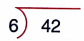Solution: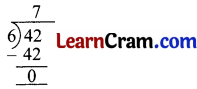(b)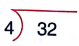Solution: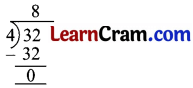(c)Solution: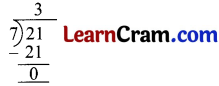(d)Solution:(e)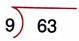Solution: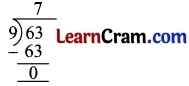(f)Solution: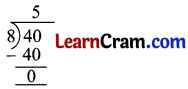(g)Solution:(h)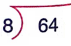Solution: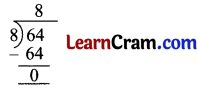Question 2.
Divide by long division method.

(a) 18 ÷ 3
Solution: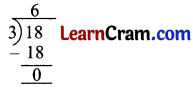(b) 54 ÷ 9
Solution: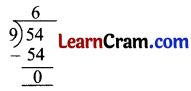(c) 36 ÷ 6
Solution:(d) 70 ÷ 10
Solution:(e) 50 ÷ 5
Solution: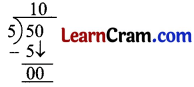(f) 32 ÷ 4
Solution:(g) 20 ÷ 10
Solution: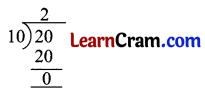(h) 28 ÷ 7
Solution: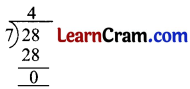### DAV Class 3 Maths Chapter 5 Worksheet 6 Notes

Long Division Method

Divide 81 by 9
81 ÷ 9 = 9
We can do the same division by another method known as long division method.
e.g.,1. Recall the table of 9.
2. Write the product of 9 and 9 un&?ath
3. Write the quotient.
4. Subtract the product from dividend.
We get zero after subtraction means the sum has no remainder.